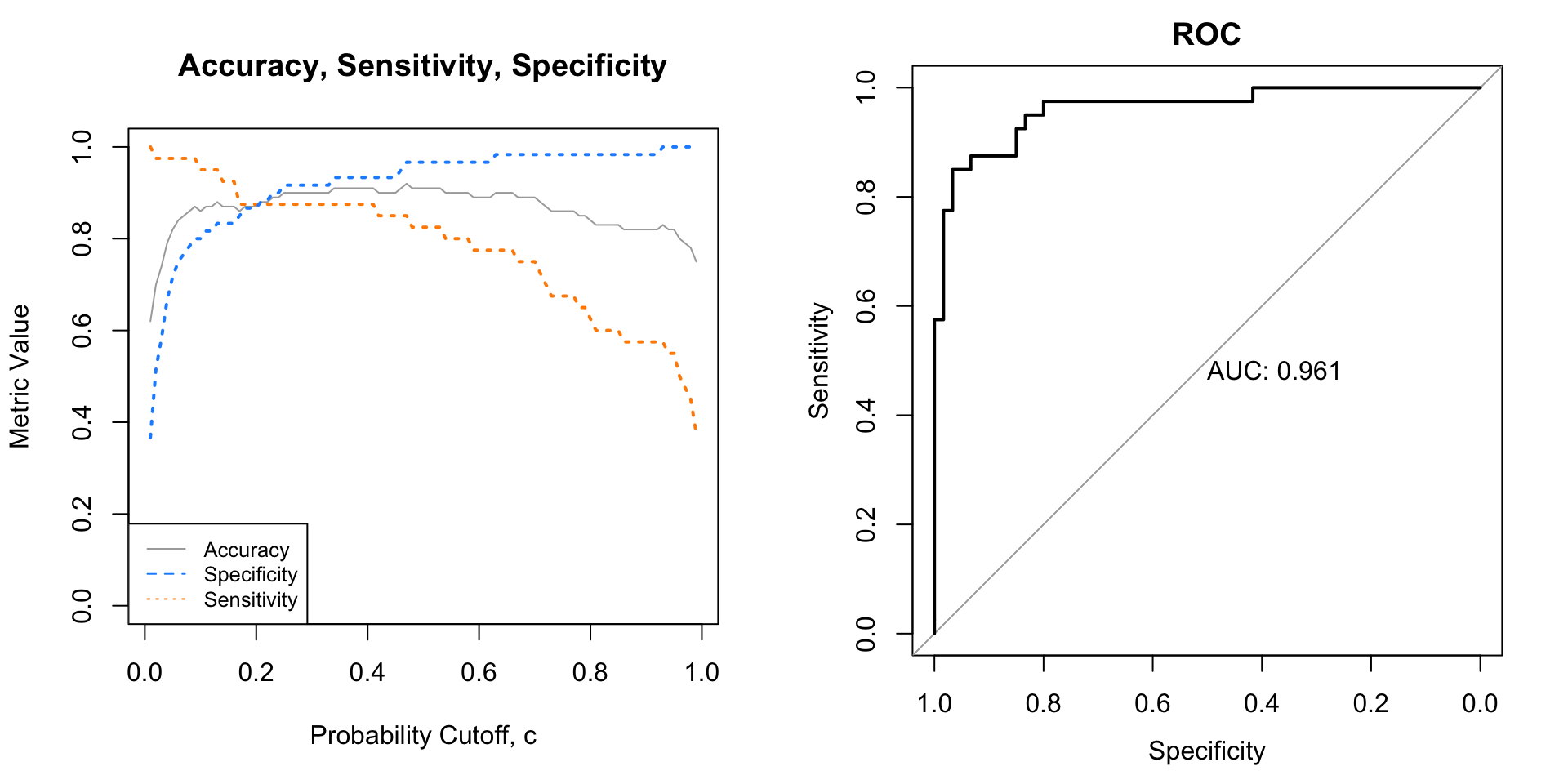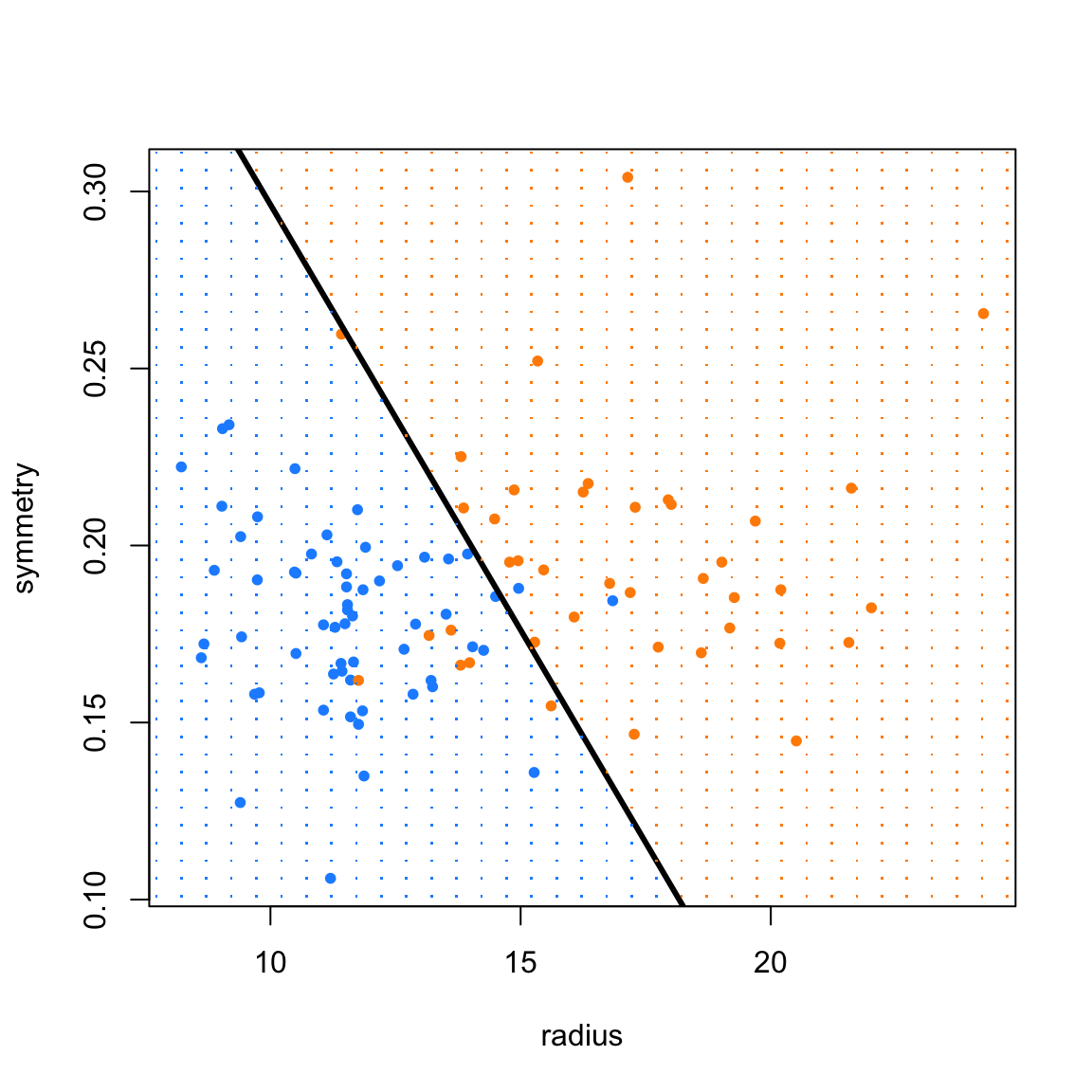For this homework, you may only use the following packages:

# general
library(caret)
library(tidyverse)
library(knitr)
library(kableExtra)

# specific
library(ISLR)
library(pROC)

# Exercise 1 (Logistic Regression for Fuel Efficiency)

[6 points] For this exercise we will use the Auto data from the ISLR package.

data(Auto)

As we have seen before, we drop the name variable. We also coerce origin and cylinders to be factors as they are categorical variables.

We also re-create a new response variable mpg. Instead of the actual fuel efficiency, we simply label cars that obtain fewer than 30 miles per gallon as ‘low’ fuel efficiency. Those above 30 have ‘high’ fuel efficiency.

Auto = subset(Auto, select = -c(name))
Auto$origin = factor(Auto$origin)
Auto$cylinders = factor(Auto$cylinders)
Auto$mpg = factor(ifelse(Auto$mpg < 30, "low", "high"))

After these modifications, we test-train split the data.

set.seed(1)
auto_trn_idx  = sample(nrow(Auto), size = trunc(0.75 * nrow(Auto)))
auto_trn_data = Auto[auto_trn_idx, ]
auto_tst_data = Auto[-auto_trn_idx, ]

The goal of our modeling in this exercise is to predict whether or not a vehicle is fuel efficient.

Fit five different logistic regressions.

• Intercept: $$\log \left( \frac{p(x)}{1 - p(x)} \right) = \beta_0$$
• Simple: $$\log \left( \frac{p(x)}{1 - p(x)} \right) = \beta_0 + \beta_1 \texttt{horsepower}$$
• Multiple:$$\log \left( \frac{p(x)}{1 - p(x)} \right) = \beta_0 + \beta_1 \texttt{horsepower} + \beta_2 \texttt{euro} + \beta_3 \texttt{japan}$$
• Interaction: An interaction model that includes all first order terms and all possible two-way interactions

Here we’ll define $$p(x) = P(Y = \texttt{low} \mid X = x)$$. The variables euro and japan are dummy variables based on the origin variables. Do not make these variables by modifying the data.

Using each of these models to estimate $$p(x)$$, that is estimate the probability of a low fuel efficiency given the characteristics of a vehicle, we can create a classifier.

$\hat{C}(x) = \begin{cases} \texttt{low} & \hat{p}(x) > 0.5 \\ \texttt{high} & \hat{p}(x) \leq 0.5 \end{cases}$

For each classifier, obtain train and test classification error rates. Summarize your results in a well-formatted markdown table.

Solution:

# fit models
mod_intercept =   glm(mpg ~ 1, data = auto_trn_data, family = "binomial")
mod_simple =      glm(mpg ~ horsepower, data = auto_trn_data, family = "binomial")
mod_multiple =    glm(mpg ~ horsepower + origin, data = auto_trn_data, family = "binomial")
mod_additive =    glm(mpg ~ ., data = auto_trn_data, family = "binomial")
mod_interaction = glm(mpg ~ . ^ 2, data = auto_trn_data, family = "binomial")
# helper function
calc_err = function(actual, predicted) {
mean(actual != predicted)
}
# create model list
auto_mod_list = list(mod_intercept, mod_simple, mod_multiple, mod_additive, mod_interaction)

# get predicted probabilities from fitted models
auto_trn_prob = lapply(auto_mod_list, predict, newdata = auto_trn_data)
auto_tst_prob = lapply(auto_mod_list, predict, newdata = auto_tst_data)

# get predictions from classifiers built using fitted models
auto_trn_pred = lapply(auto_trn_prob, function(x) {ifelse(x > 0, "low", "high")})
auto_tst_pred = lapply(auto_tst_prob, function(x) {ifelse(x > 0, "low", "high")})

# calculate errors
auto_trn_err  = sapply(auto_trn_pred, calc_err, actual = auto_trn_data$mpg) auto_tst_err = sapply(auto_tst_pred, calc_err, actual = auto_tst_data$mpg)
Model Name Train Error Test Error
Intercept 0.224 0.245
Simple 0.143 0.122
Multiple 0.136 0.102
Interaction 0.051 0.122

# Exercise 2 (Detecting Cancer with Logistic Regression)

[6 points] For this exercise we will use data found in wisc-trn.csv and wisc-tst.csv which contain train and test data respectively. wisc.csv is provided but not used. This is a modification of the Breast Cancer Wisconsin (Diagnostic) dataset from the UCI Machine Learning Repository. Only the first 10 feature variables have been provided. (And these are all you should use.)

You should consider coercing the response to be a factor variable.

Consider an additive logistic regression that considers only two predictors, radius and symmetry. Use this model to estimate

$p(x) = P(Y = \texttt{M} \mid X = x).$

Report test sensitivity, test specificity, and test accuracy for three classifiers, each using a different cutoff for predicted probability:

$\hat{C}(x) = \begin{cases} M & \hat{p}(x) > c \\ B & \hat{p}(x) \leq c \end{cases}$

• $$c = 0.1$$
• $$c = 0.5$$
• $$c = 0.9$$

We will consider M (malignant) to be the “positive” class when calculating sensitivity and specificity. Summarize these results using a single well-formatted table.

Solution:

# import data
wisc_tst = read.csv("wisc-tst.csv")
# coerce to factor
wisc_trn$class = as.factor(wisc_trn$class)
wisc_tst$class = as.factor(wisc_tst$class)
# fit model
wisc_glm = glm(class ~ radius + symmetry, data = wisc_trn, family = "binomial")
# helper function, specific to glm function
get_pred_glm = function(mod, data, res = "y", pos = 1, neg = 0, cut = 0.5) {
probs = predict(mod, newdata = data, type = "response")
ifelse(probs > cut, pos, neg)
}
# get predicted values for each cutoff
pred_10 = get_pred_glm(wisc_glm, wisc_tst, res = "class", cut = 0.1, pos = "M", neg = "B")
pred_50 = get_pred_glm(wisc_glm, wisc_tst, res = "class", cut = 0.5, pos = "M", neg = "B")
pred_90 = get_pred_glm(wisc_glm, wisc_tst, res = "class", cut = 0.9, pos = "M", neg = "B")
# create table for each cutoff
tab_10 = table(predicted = pred_10, actual = wisc_tst$class) tab_50 = table(predicted = pred_50, actual = wisc_tst$class)
tab_90 = table(predicted = pred_90, actual = wisc_tst$class) # coerce tables to be confusion matrixes con_mat_10 = confusionMatrix(tab_10, positive = "M") con_mat_50 = confusionMatrix(tab_50, positive = "M") con_mat_90 = confusionMatrix(tab_90, positive = "M") metrics = rbind( c(con_mat_10$overall["Accuracy"],
con_mat_10$byClass["Sensitivity"], con_mat_10$byClass["Specificity"]),
c(con_mat_50$overall["Accuracy"], con_mat_50$byClass["Sensitivity"],
con_mat_50$byClass["Specificity"]), c(con_mat_90$overall["Accuracy"],
con_mat_90$byClass["Sensitivity"], con_mat_90$byClass["Specificity"])
)

rownames(metrics) = c("c = 0.10", "c = 0.50", "c = 0.90")
kable_styling(kable(metrics, format = "html", digits = 3), full_width = FALSE)
Accuracy Sensitivity Specificity
c = 0.10 0.86 0.950 0.800
c = 0.50 0.91 0.825 0.967
c = 0.90 0.82 0.575 0.983

# Exercise 3 (More Sensitivity and Specificity)

[6 points] Continuing the setup (data and model) from Exercise 2, we now create two plots which will help us understand the tradeoff between sensitivity and specificity.

• Plot 1 A plot that shows (test) accuracy, sensitivity, and specificity as a function of the cutoff $$c$$ used to create a classifier based on the logistic regression model.
• Use the test data.
• Consider values of c from 0.01 to 0.99. (See R code below.)
• Accuracy, sensitivity, and specificity will each be a “line” on the plot.
• Give each line a different color and line type.
• Give the plot a title, axis labels, and legend.
• Plot 2 An ROC Curve
• Use the test data.
• Display the AUC value on the plot.

Display these two plots side-by-side.

Hint: Consider creating some functions specific to this exercise for obtaining accuracy, sensitivity, and specificity. (If you didn’t already do so in Exercise 2.)

c = seq(0.01, 0.99, by = 0.01)

Solution:

get_metric = function(c, metric) {
pred = get_pred_glm(wisc_glm, wisc_tst, res = "class", cut = c, pos = "M", neg = "B")
tab = table(predicted = pred, actual = wisc_tst$class) conmat = confusionMatrix(tab, positive = "M") ifelse(metric == "acc", conmat$overall["Accuracy"],
ifelse(metric == "sens", conmat$byClass["Sensitivity"], conmat$byClass["Specificity"]))
}
# setup for custom plotting
wisc_acc  = sapply(c, get_metric, metric = "acc")
wisc_sens = sapply(c, get_metric, metric = "sens")
wisc_spec = sapply(c, get_metric, metric = "spec")

# setup for ROC
test_prob = predict(wisc_glm, newdata = wisc_tst, type = "response")
par(mfrow = c(1, 2))

# custom plot
plot(c, wisc_acc, typ = "l", ylim = c(0, 1), col = "darkgrey", lwd = 1, lty = 1,
main = "Accuracy, Sensitivity, Specificity",
xlab = "Probability Cutoff, c", ylab = "Metric Value")
lines(c, wisc_sens, col = "darkorange", lwd = 2, lty = 3)
lines(c, wisc_spec, col = "dodgerblue", lwd = 2, lty = 3)
legend("bottomleft", c("Accuracy", "Specificity", "Sensitivity"), lty = c(1, 2, 3),
lwd = 1, col = c("darkgrey", "dodgerblue", "darkorange"), cex = 0.8)

# ROC plot
test_roc = roc(wisc_tst\$class ~ test_prob, plot = TRUE, print.auc = TRUE,
main = "ROC")# Exercise 4 (Logistic Regression Decision Boundary)

[6 points] Continue with the cancer data from previous exercises. Again, consider an additive logistic regression that considers only two predictors, radius and symmetry. Plot the test data with radius as the $$x$$ axis, and symmetry as the $$y$$ axis, with the points colored according to their tumor status. Add a line which represents the decision boundary for a classifier using 0.5 as a cutoff for predicted probability.

Solution:

wisc_glm = glm(class ~ radius + symmetry, data = wisc_trn, family = "binomial")
# function to calculate decision boundary for glms
glm_boundary_line = function(glm_fit) {
intercept = as.numeric(-coef(glm_fit) / coef(glm_fit))
slope = as.numeric(-coef(glm_fit) / coef(glm_fit))
c(intercept = intercept, slope = slope)
}
# function to add decision boundary for glms to an existing plot
add_glm_boundary = function(glm_fit, line_col = "black") {
abline(glm_boundary_line(glm_fit), col = line_col, lwd = 3)
}# Exercise 5 (Concept Checks)

[1 point each] Answer the following questions based on your results from the three exercises.

(a) What is $$\hat{\beta}_2$$ for the multiple model in Exercise 1?

Solution:

coef(mod_multiple)
##   origin2
## 0.1243737

(b) Based on your results in Exercise 1, which of these models performs best?

Solution: The additive model achieves the lowest test error.

(c) Based on your results in Exercise 1, which of these models do you think may be underfitting?

Solution: The intercept, simple, and multiple models as they all simpler than the best model, with more error.

(d) Based on your results in Exercise 1, which of these models do you think may be overfitting??

Solution: The interaction model as it is more complex than the best model, with more error.

(e) Of the classifiers in Exercise 2, which do you prefer?

Solution: The model with c = 0.10.

(f) State the metric you used to make your decision in part (e), and a reason for using that metric.

Solution: The model with c = 0.10 achieves the highest sensitivity, meaning it most oftens labels cancer as cancer! We value this over labeling non-cancer as non-cancer.